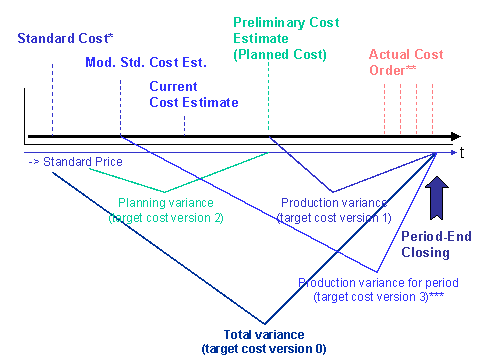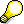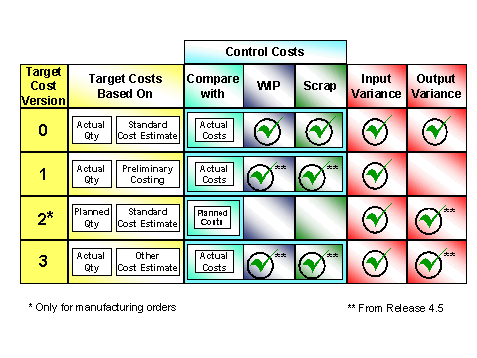Types of Variance CalculationYou can calculate the following variances in the R/3 system:

• Planning variances
• Production variances
• Production variance of the period
• Total variance

You control the variance calculation process by specifying a particular target cost version.* In make-to-stock production, standard cost is calculated in the standard cost estimate for the material. In sales-order-related production with a valuated sales order stock, standard cost is determined using a predefined valuation strategy.

** During production, actual costs are collected on the order (product cost collector or manufacturing order). The actual costs that are compared with the target costs are reduced by the work in process and scrap variances (the result is called the net actual cost).

*** You can determine the production variances of the period by comparing an alternative material cost estimate with the (net) actual costs. This alternative material cost estimate can be the modified standard cost estimate or the current cost estimate, for example.

Total Variance

The total variance is the only variance that is relevant to settlement. This means that only the variance categories of target cost version 0 can be transferred to Profitability Analysis (CO-PA).

The total variance is the difference between the actual costs debited to the order and the costs credited to the order due to deliveries to stock. The difference between the debit and credit of the order is passed to Financial Accounting (FI) and Profit Center Accounting (EC-PCA) when the order is settled.

The target costs for the total variance are calculated as follows:

• In make-to-stock production, the target costs are calculated using the standard cost estimate for the material and the quantity delivered to stock.
• In sales-order-related production with a valuated sales order stock, the target costs are calculated using the cost estimate relevant to the valuation of the sales order stock (such as a sales order cost estimate or a standard cost estimate for the manufacturing order) and the quantity delivered to stock.

For the total variance, the control costs are equivalent to the following:

• In Product Cost by Order, the control costs are the actual costs less the scrap variances.
• In Product Cost by Period, the control costs are the actual costs less the work in process and scrap variances.

The variance calculation process compares the itemization of the cost estimate on which the calculation of target costs is based with the line items in your actuals.

The itemization contains detailed information based on the costing lot size, such as the materials to be used and the activities to be performed.

When you release a standard cost estimate for a material, the price calculated therein becomes the standard price in the material master record of the material being manufactured. In the standard system, an itemization is always created for standard cost estimates for materials.

The following variance categories can be reported for the total variance:

• Input price variance
• Resource-usage variance
• Input quantity variance
• Remaining input variance
• Scrap variance
• Mixed-price variance
• Output price variance
• Lot size variance
• Remaining variance

When you settle, you can transfer the total variance to Profitability Analysis (CO-PA) broken down into variance categories.

In CO-PA, the standard cost of goods manufactured for the product is compared with the revenues to calculate an initial contribution margin for the period. The variances between actual cost and standard cost of goods manufactured can be used to calculate a second contribution margin for the period.

When you calculate the variances, you can transfer the difference between the debit and credit to CO-PA only as a total.

You calculate the total variance with target cost version 0.

Special Features with a Valuated Sales Order Stock

If you are using a valuated sales order stock, the sales order stock is valuated according to the settings in Customizing for the Product Cost by Sales Order component in the Requirements Class.If you are using a valuated sales order stock, you always create a sales order cost estimate with an itemization when you want to calculate work in process, variances, and scrap variances on the basis of that cost estimate.

No itemization is available if the sales order stock is valuated on the basis of a preliminary cost estimate for the production order or process order. In this case the itemization is determined dynamically when the total variance is calculated.This may have adverse effects on performance.

In your actuals, the line items for the production order contain detailed information for the order, such as the materials used and the activities performed.

Production Variances

For production variances, the target costs are based on the preliminary cost estimate for the manufacturing order or product cost collector and the quantity delivered to stock.

For production variances, the control costs are the net actual costs and depend on the variance variant.

This determines the variances that occurred between the creation of the production order and the completion of the production process.

The system assigns the production variances to variance categories.

In the standard system the production variance is calculated with target cost version 1. Target cost version 1 can also be used for the calculation of equivalences for the distribution of actual costs in cost object hierarchies. Target cost version 1 is not relevant to settlement.

When you determine the production variances for the product cost collector, with target cost version 1 you calculate the target costs on the basis of the preliminary cost estimate for the product cost collector. If you are using a product cost collector, the target costs cannot be determined on the basis of the preliminary cost estimate for the manufacturing order.

The following variance categories can be calculated for production variances:

• Input price variance
• Resource-usage variance
• Input quantity variance
• Remaining input variance

Production Variance Against Planned Price of Period

To calculate the planned price for the period, create an alternative material cost estimate (such as a modified standard cost estimate) in each period. The target costs are calculated using this alternative material cost estimate and the yield. The control costs are the net actual costs and depend on the variance variant.

This enables you to determine the production variances between the planned price of the period and the actual costs incurred during the production process.

In the standard system the production variance of the period is calculated with target cost version 3. Target cost version 3 can also be used for the calculation of equivalences for the distribution of actual costs in cost object hierarchies. Target cost version 3 is not relevant to settlement.

The following variance categories can be calculated for the production variances of the period:

• Input price variance
• Resource-usage variance
• Input quantity variance
• Remaining input variance
• Scrap variance
• Mixed-price variance
• Output price variance
• Lot size variance

Planning Variances

For planning variances, the target costs are based on the standard cost estimate for the material and the planned order quantity.

The control costs equal the costs calculated in the preliminary cost estimate for the order.

When you create a production order or process order, the planned costs for the order are automatically calculated if you enter a costing variant in the order header.

This determines the variances that occurred between the creation of the standard cost estimate and the creation of the production order or process order. You can therefore determine which variances occurred due to changes to the BOM or routing. If there are significant lot size variances, for example, you may want to group multiple order lots together.

In the standard system you calculate the planning variances with target cost version 2. Target cost version 2 is not relevant to settlement.

The following variance categories can be calculated for planning variances:

• Input price variance
• Resource-usage variance
• Input quantity variance
• Remaining input variance
• Scrap variance

You cannot calculate a planning variance between a current standard cost estimate and a preliminary cost estimate for the product cost collector.

If you are using a valuated sales order stock, you cannot calculate a planning variance if the standard price was calculated in the preliminary cost estimate for the manufacturing order.Note that in most cases the total variance is not the sum of the production variance and the planning variance. The formula Total variance (target cost version 0) = Production variance (target cost version 1) + Planning variance (target cost version 2) only applies if target cost version 2 is adjusted to the same allocation base as target cost versions 0 and 1. This means that the yield quantity must be the same as the planned order quantity. In practice this will only happen in exceptional cases.

Customizing for Variance Calculation: Target Cost Versions